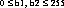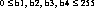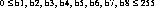`Packages  This Package  Prev  Next  Index  `

# §2.5 Class DataInputStream

```public  class  java.io.DataInputStream
extends  java.io.FilterInputStream  (I-§2.11)
implements java.io.DataInput  (I-§2.24)
{
// Constructors
public DataInputStream(InputStream  in);	§2.5.1

// Methods
public final int read(byte  b[]);	§2.5.2
public final int read(byte  b[], int  off, int  len);	§2.5.3
public final void readFully(byte  b[]);	§2.5.9
public final void readFully(byte  b[], int  off, int  len);	§2.5.10
public final static String readUTF(DataInput  in);	§2.5.18
public final int skipBytes(int  n);	§2.5.19
}
```
A data input stream lets an application read primitive Java data types from an underlying input stream in a machine-independent way. An application uses a data output stream (I-§2.6) to write data which can later be read by a data input stream.

Data input streams and data output streams represent Unicode strings in a format that is a slight modification of UTF-81 All characters in the range '\u0001' to '\u007F' are represented by a single byte:
 0 bits 0-7

The null character '\u0000' and characters in the range '\u0080' to '\u07FF' are represented by a pair of bytes:
 1 1 0 bits 6-10 1 0 bits 0-5

Characters in the range '\u0800' to '\uFFFF' are represented by three bytes:
 1 1 1 0 bits 12-15 1 0 bits 6-11 1 0 bits 0-5

The two differences between this format and the "standard" UTF-8 format are the following:

##### DataInputStream

```public DataInputStream(InputStream in) ```
Creates a new data input stream to read data from the specified input stream.
Parameters:
```in - ```the input stream

##```public final int read(byte b[]) ``` ```throws IOException ```
Reads up to byte.length bytes of data from this data input stream into an array of bytes. This method blocks until some input is available.
The read method of DataInputStream calls the the read method (I-§2.13.8) of its underlying input stream (I-§2.11.1) with the three arguments b, 0, and b.length, and returns whatever value that method returns.

Parameters:
```b - ```the buffer into which the data is read
Returns:
the total number of bytes read into the buffer, or -1 is there is no more data because the end of the stream has been reached.
Throws
IOException (I-§2.29)
If an I/O error occurs.
Overrides:

```public final int read(byte b[], int off, int len) ``` ```throws IOException ```
Reads up to len bytes of data from this data input stream into an array of bytes. This method blocks until some input is available.
The read method of DataInputStream calls the read method (I-§2.13.8) of its underlying input stream (I-§2.11.1) with the same arguments and returns whatever value that method returns.

Parameters:
```b - ```the buffer into which the data is read
```off - ```the start offset of the data
```len - ```the maximum number of bytes read
Returns:
the total number of bytes read into the buffer, or -1 is there is no more data because the end of the stream has been reached.
Throws
IOException (I-§2.29)
If an I/O error occurs.
Overrides:

```public final boolean readBoolean() ``` ```throws IOException ```
Reads a boolean from this data input stream. This method reads a single byte from the underlying input stream (I-§2.11.1). A value of 0 represents false. Any other value represents true. This method blocks until either the byte is read, the end of the stream is detected, or an exception is thrown.

Returns:
Throws
EOFException (I-§2.24)
If this input stream has reached the end.
Throws
IOException (I-§2.29)
If an I/O error occurs.

```public final byte readByte() ``` ```throws IOException ```
Reads a signed 8-bit value from this data input stream. This method reads a byte from the underlying input stream (I-§2.11.1). If the byte read is b, where, then the result is

```public final char readChar() ``` ```throws IOException ```
Reads a Unicode character from this data input stream. This method reads two bytes from the underlying input stream (I-§2.11.1). If the bytes read, in order, are b1 and b2, where, then the result is equal to

```public final double readDouble() ``` ```throws IOException ```
Reads a double from this data input stream. This method reads a long value as if by the readLong method (I-§2.5.13) and then converts that long to a double using the long-Bits-To-Double method (I-§1.6.18) in class Double.

This method blocks until either the eight bytes are read, the end of the stream is detected, or an exception is thrown.
Returns:
the next eight bytes of this input stream, interpreted as a double.
Throws
EOFException (I-§2.24)
If this input stream reaches the end before reading eight bytes.
Throws
IOException (I-§2.29)
If an I/O error occurs.

```public final float readFloat() ``` ```throws IOException ```
Reads a float from this data input stream. This method reads an int value as if by the readInt method (I-§2.5.11) and then converts that int to a float using the intBitsToFloat method (I-§1.7.14) in class Float. This method blocks until either the four bytes are read, the end of the stream is detected, or an exception is thrown.

Returns:
the next four bytes of this input stream, interpreted as a float.
Throws
EOFException (I-§2.24)
If this input stream reaches the end before reading four bytes.
Throws
IOException (I-§2.29)
If an I/O error occurs.

```public final void readFully(byte b[]) ``` ```throws IOException ```
Reads b.length bytes from this data input stream into the byte array. This method reads repeatedly from the underlying stream (I-§2.11.1) until all the bytes are read. This method blocks until either all the bytes are read, the end of the stream is detected, or an exception is thrown.

Parameters:
```b - ```the buffer into which the data is read
Throws
EOFException (I-§2.24)
If this input stream reaches the end before reading all the bytes.
Throws
IOException (I-§2.29)
If an I/O error occurs.

```public final void readFully(byte b[], int off, int len) ``` ```throws IOException ```
Reads exactly len bytes from this data input stream into the byte array. This method reads repeatedly from the underlying stream (I-§2.11.1) until all the bytes are read. This method blocks until either all the bytes are read, the end of the stream is detected, or an exception is thrown.

Parameters:
```b - ```the buffer into which the data is read
```off - ```the start offset of the data
```len - ```the number of bytes to read read
Throws
EOFException (I-§2.24)
If this input stream reaches the end before reading all the bytes.
Throws
IOException (I-§2.29)
If an I/O error occurs.

```public final int readInt() ``` ```throws IOException ```
Reads a signed 32-bit integer from this data input stream. This method reads four bytes from the underlying input stream (I-§2.11.1). If the bytes read, in order, are b1, b2, b3, and b4 where, then the result is equal to

```public final String readLine() ``` ```throws IOException ```
Reads the next line of text from this data input stream. This method successively reads bytes from the underlying input stream (I-§2.11.1) until it reaches the end of a line of text.

A line of text is terminated by a carriage return character ('\r'), a newline character ('\n'), a carriage return character immediately followed by a newline character, or the end of the input stream. The line-terminating character(s), if any, are included as part of the string returned.
This method blocks until either a newline character is read, a carriage return and the byte following it are read (to see if it is a newline), the end of the stream is detected, or an exception is thrown.
Returns:
the next line of text from this input stream.
Throws
IOException (I-§2.29)
If an I/O error occurs.

```public final long readLong() ``` ```throws IOException ```
Reads a signed 64-bit integer from this data input stream. This method reads eight bytes from the underlying input stream (I-§2.11.1). If the bytes read, in order, are b1, b2, b3, b4, b5, b6, b7, and b8, where,
then the result is equal to

```public final short readShort() ``` ```throws IOException ```
Reads a signed 16-bit number from this data input stream. The method reads two bytes from the underlying input stream (I-§2.11.1). If the two bytes read, in order, are b1 and b2, where each of the two values is between 0 and 255, inclusive, then the result is equal to:

(short)((b1 << 8) | b2)
This method blocks until either the two bytes are read, the end of the stream is detected, or an exception is thrown.
Returns:
the next two bytes of this input stream, interpreted as a signed 16-bit number.
Throws
EOFException (I-§2.24)
If this input stream reaches the end before reading two bytes.
Throws
IOException (I-§2.29)
If an I/O error occurs.

```public final int readUnsignedByte() ``` ```throws IOException ```
Reads an unsigned 8-bit number from this data input stream. This method reads a byte from this data input stream's underlying input stream (I-§2.11.1) and returns that byte This method blocks until either the byte is are read, the end of the stream is detected, or an exception is thrown.

Returns:
the next byte of this input stream, interpreted as an unsigned 8-bit number.
Throws
EOFException (I-§2.24)
If this input stream has reached the end.
Throws
IOException (I-§2.29)
If an I/O error occurs.

```public final int readUnsignedShort() ``` ```throws IOException ```
Reads an unsigned 16-bit number from this data input stream. This method reads two bytes from the underlying input stream (I-§2.11.1). If the bytes read, in order, are b1 and b2, where, then the result is equal to

```public final String readUTF() ``` ```throws IOException ```
Reads in a string which has been encoded using a modified UTF-8 format from this data input stream. This method calls readUTF(this). See the following method for a more complete description of the format.
This method blocks until either all the bytes are read, the end of the stream is detected, or an exception is thrown.
Returns:
a Unicode string.
Throws
EOFException (I-§2.24)
If this input stream reaches the end before reading all the bytes.
Throws
IOException (I-§2.29)
If an I/O error occurs.

```public final static String readUTF(DataInput in) ``` ```throws IOException ```
Reads in a string from the specified data input stream. The string has been encoded using a modified using a modified UTF-8 format.
The first two bytes from are read as if by readUnsignedShort (I-§2.5.16). This values gives the number of following bytes that are in the encoded string. (Note: not the length of the resuling string). The following bytes are then interpreted as bytes encoding characters in the UTF-8 format (page I-225) and are converted into characters.

This method blocks until all the bytes are read, the end of the stream is detected, or an exception is thrown.
Parameters:
```in - ```a data input stream
Returns:
a Unicode string.
Throws
EOFException (I-§2.24)
If the input stream reaches the end before all the bytes.
Throws
UTFDataFormatException (I-§2.31)
If the bytes do not represent a valid UTF-8 encoding of a Unicode string.
Throws
IOException (I-§2.29)
If an I/O error occurs.

### skipBytes

```public final int skipBytes(int n) ``` ```throws IOException ```
Skips exactly n bytes of input in the underlying input stream (I-§2.11.1). This method blocks until either all the bytes are skipped, the end of the stream is detected, or an exception is thrown.

Parameters:
```n - ```the number of bytes to be skipped
Returns:
the number of bytes skipped, which is always n.
Throws
EOFException (I-§2.24)
If this input stream reaches the end before skipping all the bytes.
Throws
IOException (I-§2.29)
If an I/O error occurs.

1 X/Open Company Ltd., "File System Safe UCS Transformation Format (FSS_UTF)", X/Open Preliminary Specification, Document Number: P316. This information also appears in ISO/IEC 10646, Annex P.

`Packages  This Package  Prev  Next  Index`
Java API Document (HTML generated by dkramer on April 22, 1996)